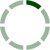### Sort by Date ▼ / Top Rated

Costco Interest Expense
For TGT and WMT, interest expense was taken as the gross total (including interest income). But, for COST only interest expense is included. Should that number be 144 instead of 34? (143.533 rounded, which is the interest expense (34,437) and interest income (109,096) summed to get gross interest)
Averaging When Mixing IS and BS Items
It was my understanding, through both the video and the footnotes on the slides, that we were to average inventory, A/R and A/P balances for the Days Outstanding Ratios. When is it applicable to average and when are the exceptions? Thank you!
0
Current liabilities
Hi, one question, would it be correct to exclude the interest-bearing liabilities when calculating the liquidity ratios? I've seen it a lot and just wanted to hear your opinion. Thanks!
0
Current Liabilities
When we calculate the liquidity ratios, would it make sense to exclude the short-term interesting-bearing liabilities from the current liabilities? So, e.g., instead of using \$48,826 for WMT, we could use \$40,178. I've seen this done a couple of times and wanted to make sure what is the right way. S... Read More
0
Year of statements inquiry
Please tell me why do you use all the numbers in the video from the year 2006, while on the table grid when have year 2005 ?!